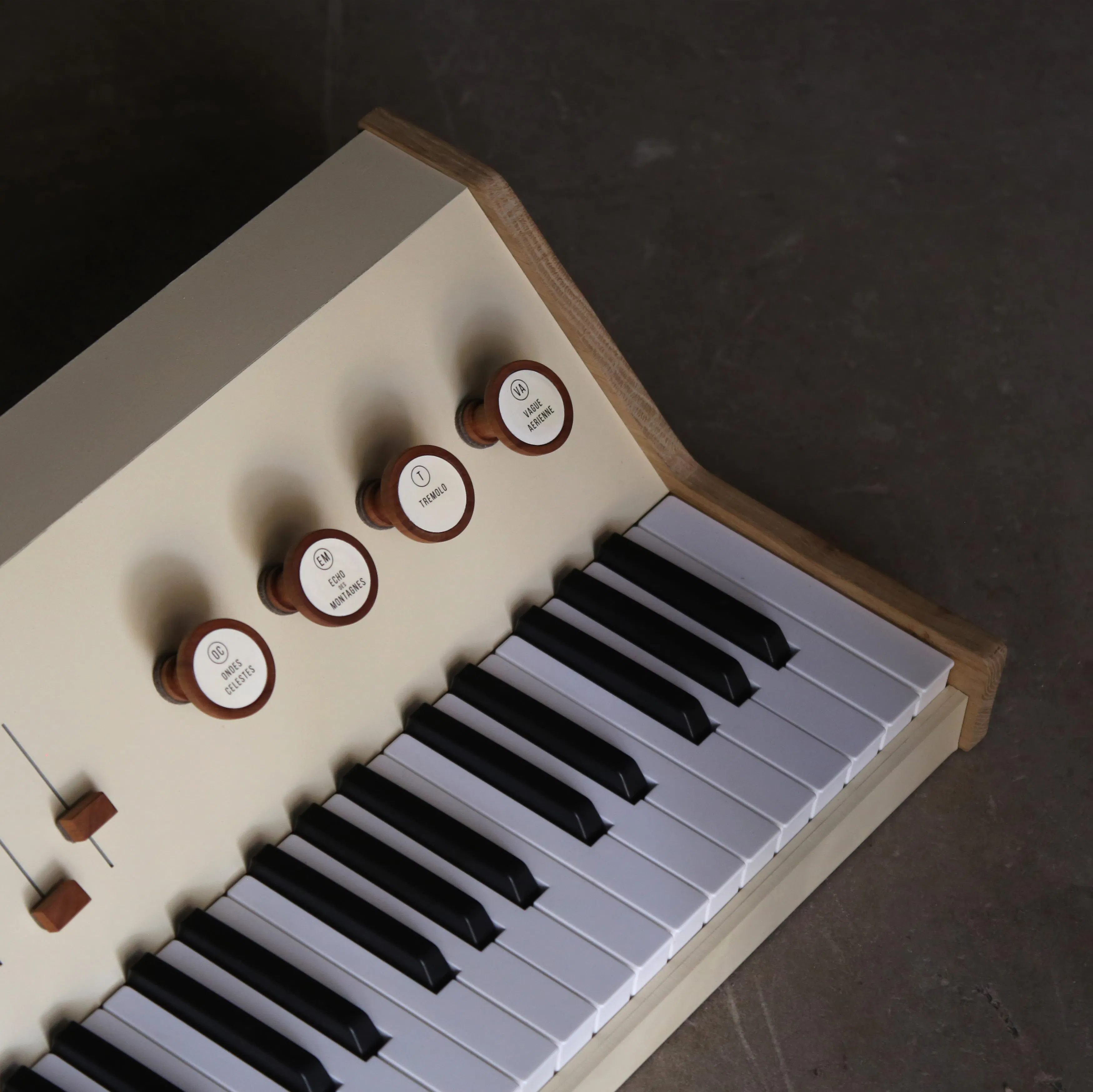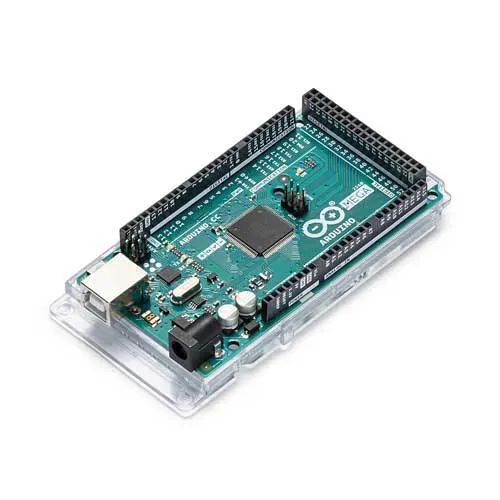Published © CC BY

# Pianographe

My brother and I created a midi synthetiser called the Pianographe.

IntermediateShowcase (no instructions)6,730## Things used in this project

### Hardware componentsArduino Mega 2560
×1

Read more

## Code

### Pianographe

Arduino
```int val = 0; //Our initial pot values. We need one for the first value and a second to test if there has been a change made. This needs to be done for all 3 pots.
int lastVal = 0;
int val2 = 0;
int lastVal2 = 0;
int val3 = 0;
int lastVal3 = 0;
int val4 = 0;
int lastVal4 = 0;
int val5 = 0;
int lastVal5 = 0;
int val6 = 0;
int lastVal6 = 0;
int val7 = 0;
int lastVal7 = 0;
int val8 = 0;
int lastVal8 = 0;
int val9 = 0;
int lastVal9 = 0;
int val10 = 0;
int lastVal10 = 0;
int val11 = 0;
int lastVal11 = 0;
int val12 = 0;
int lastVal12 = 0;

void setup()
{
Serial.begin(9600);       // Set the speed of the midi port to the same as we will be using in the Hairless Midi software
}

void loop()
{
val = analogRead(0)/8;   // Divide by 8 to get range of 0-127 for midi
if (val != lastVal) // If the value does not = the last value the following command is made. This is because the pot has been turned. Otherwise the pot remains the same and no midi message is output.
{
MIDImessage(176,1,val);}         // 176 = CC command (channel 1 control change), 1 = Which Control, val = value read from Potentionmeter 1 NOTE THIS SAYS VAL not VA1 (lowercase of course)
lastVal = val;

val2 = analogRead(1)/8;   // Divide by 8 to get range of 0-127 for midi
if (val2 != lastVal2)
{
MIDImessage(176,2,val2);}         // 176 = CC command, 2 = Which Control, val = value read from Potentionmeter 2
lastVal2 = val2;

val3 = analogRead(2)/8;   // Divide by 8 to get range of 0-127 for midi
if (val3 != lastVal3)
{
MIDImessage(176,3,val3);}         // 176 = CC command, 3 = Which Control, val = value read from Potentionmeter 3
lastVal3 = val3;

val4 = analogRead(3)/8;   // Divide by 8 to get range of 0-127 for midi
if (val4 != lastVal4)
{
MIDImessage(176,4,val4);}         // 176 = CC command, 3 = Which Control, val = value read from Potentionmeter 3
lastVal4 = val4;

val5 = analogRead(4)/8;   // Divide by 8 to get range of 0-127 for midi
if (val5 != lastVal5)
{
MIDImessage(176,5,val5);}         // 176 = CC command, 3 = Which Control, val = value read from Potentionmeter 3
lastVal5 = val5;

val6 = analogRead(5)/8;   // Divide by 8 to get range of 0-127 for midi
if (val6 != lastVal6)
{
MIDImessage(176,6,val6);}         // 176 = CC command, 3 = Which Control, val = value read from Potentionmeter 3
lastVal6 = val6;

val7 = analogRead(6)/8;   // Divide by 8 to get range of 0-127 for midi
if (val7 != lastVal7)
{
MIDImessage(176,7,val7);}         // 176 = CC command, 3 = Which Control, val = value read from Potentionmeter 3
lastVal7 = val7;

val8 = analogRead(7)/8;   // Divide by 8 to get range of 0-127 for midi
if (val8 != lastVal8)
{
MIDImessage(176,8,val8);}         // 176 = CC command, 3 = Which Control, val = value read from Potentionmeter 3
lastVal8 = val8;

val9 = analogRead(8)/8;   // Divide by 8 to get range of 0-127 for midi
if (val9 != lastVal9)
{
MIDImessage(176,9,val9);}         // 176 = CC command, 3 = Which Control, val = value read from Potentionmeter 3
lastVal9 = val9;

val10 = analogRead(9)/8;   // Divide by 8 to get range of 0-127 for midi
if (val10 != lastVal10)
{
MIDImessage(176,10,val10);}         // 176 = CC command, 3 = Which Control, val = value read from Potentionmeter 3
lastVal10 = val10;

val11 = analogRead(10)/8;   // Divide by 8 to get range of 0-127 for midi
if (val11 != lastVal11)
{
MIDImessage(176,11,val11);}         // 176 = CC command, 3 = Which Control, val = value read from Potentionmeter 3
lastVal11 = val11;

val12 = analogRead(11)/8;   // Divide by 8 to get range of 0-127 for midi
if (val12 != lastVal12)
{
MIDImessage(176,12,val12);}         // 176 = CC command, 3 = Which Control, val = value read from Potentionmeter 3
lastVal12 = val12;
delay(15); //here we add a short delay to help prevent slight fluctuations, knocks on the pots etc. Adding this helped to prevent my pots from jumpin up or down a value when slightly touched or knocked.
}

void MIDImessage(byte command, byte data1, byte data2) //pass values out through standard Midi Command
{
Serial.write(command);
Serial.write(data1);
Serial.write(data2);
}
```

## Credits

### idioma

1 project • 6 followers